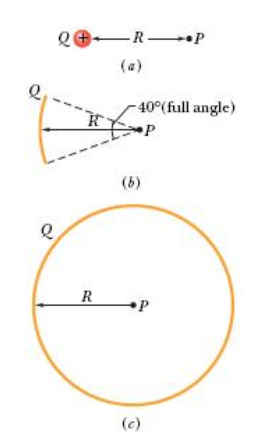# Problem: Answer the following questions about the charge distributions shown below.  Part A. In Fig. 24-28a, what is the potential at point P due to charge Q at distance R from P? Set V = O at infinity, Express your answer in terms of given variables, ε0 and π.Part B. In Fig. 24-28b, the same charge Q has been spread uniformly over a circular arc of radius R and central angle 40°. What is the potential at point P, the center of curvature of the arc? Express your answer in terms of given variables ε0 and π.Part C. In Fig. 24-28c, the same charge Q has been spread uniformly over a circle of radius R. What is the potential at point P, the center of the circle? Express your answer in terms of given variables, ε0 and π. Part D. Rank the three situations according to the magnitude of the electric field that is set up at P, greatest first. If multiple situations rank equally, use the same rank for each, then exclude the intermediate ranking (.e. if objects A, B, and C must be ranked, and A and B must both be ranked first, the ranking would be A:1, B:1, C:3). If all sluations rank equally, rank each as 1

###### FREE Expert Solution

The electric potential at point P due to charge Q is expressed as:

$\overline{){{\mathbf{V}}}_{{\mathbf{P}}}{\mathbf{=}}\frac{\mathbf{k}\mathbf{Q}}{\mathbf{r}}}$

96% (32 ratings)###### Problem Details

Answer the following questions about the charge distributions shown below.Part A. In Fig. 24-28a, what is the potential at point P due to charge Q at distance R from P? Set V = O at infinity, Express your answer in terms of given variables, ε0 and π.

Part B. In Fig. 24-28b, the same charge Q has been spread uniformly over a circular arc of radius R and central angle 40°. What is the potential at point P, the center of curvature of the arc? Express your answer in terms of given variables ε0 and π.

Part C. In Fig. 24-28c, the same charge Q has been spread uniformly over a circle of radius R. What is the potential at point P, the center of the circle? Express your answer in terms of given variables, ε0 and π.

Part D. Rank the three situations according to the magnitude of the electric field that is set up at P, greatest first. If multiple situations rank equally, use the same rank for each, then exclude the intermediate ranking (.e. if objects A, B, and C must be ranked, and A and B must both be ranked first, the ranking would be A:1, B:1, C:3). If all sluations rank equally, rank each as 1

Frequently Asked Questions

What scientific concept do you need to know in order to solve this problem?

Our tutors have indicated that to solve this problem you will need to apply the Electric Potential concept. You can view video lessons to learn Electric Potential. Or if you need more Electric Potential practice, you can also practice Electric Potential practice problems.

What professor is this problem relevant for?

Based on our data, we think this problem is relevant for Professor Borvak's class at University of Dallas.